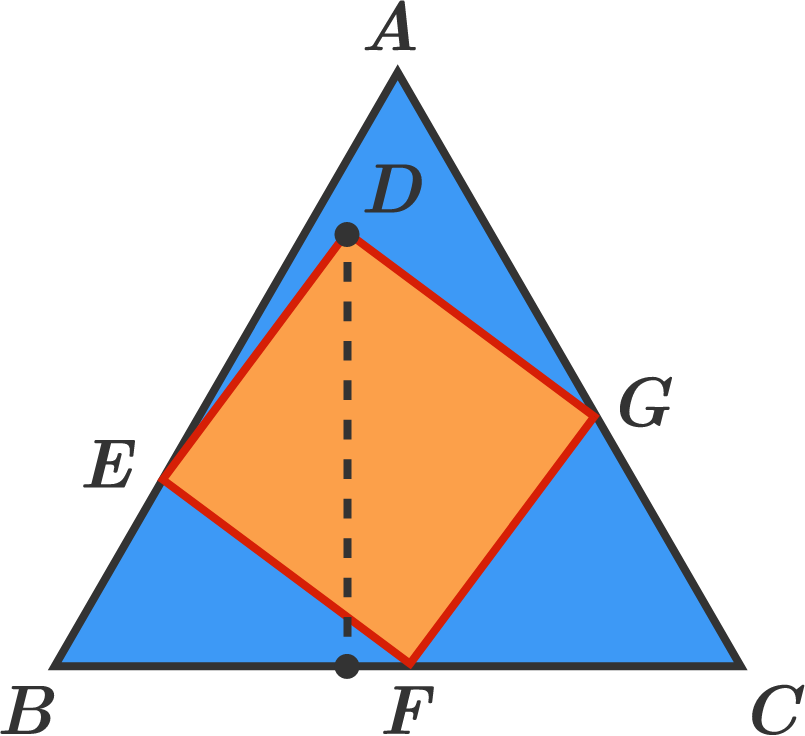# Find the length of the perpendicular to the base of this equilateral triangle.

Geometry Level 2

$ABC$ is an equilateral triangle with side length 1. Square $DEFG$ is placed in triangle $ABC$ such that points $E,$ $F,$ and $G$ are on segments $AB,$ $BC,$ and $CA,$ respectively.

What is the length of the perpendicular from point $D$ to segment $BC?$×